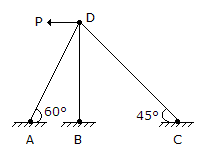# Civil Engineering - Theory of Structures - Discussion

Discussion Forum : Theory of Structures - Section 4 (Q.No. 16)
16.
The degree of indeterminacy of the frame in the given figure, iszero
1
2
3
Explanation:
No answer description is available. Let's discuss.
Discussion:
15 comments Page 1 of 2.

Manoj Pakur said:   2 weeks ago
For frame;
3(m-j)+r-c
Here,
m=3
J=4
r=6
C=2
Solving, degree of indeterminacy = q.
(1)

B.Singh said:   1 year ago
Degree of external indeterminacy = r - 3 = 6 - 3 = 3.
Degree of internal indeterminacy = m - (2j - 3) = 3 - (2*4 - 3) = -2,
Degree of static indeterminacy = External + Internal = 3 -2 = 1.

Alternatively,
Degree of static indeterminacy = (m + r) - 2j = (3 + 6) - 2 * 4 = 1.
(1)

Jaydip tiwari said:   2 years ago
I think M+r-2j is used for Truss not for FRAME.

Muhammad usman said:   2 years ago
Since it is frame no of the internal loop is zero the reaction and the point where the load is acting like hinge then total indeterminacy is equal to external +internal.

External=number of reaction - equilibrium equation=6-3=3.
Internal=3c-rection realized by a hinge.

Here c=number of closed loop = 0.
Rr=reaction released by hinge = sum (m-1).

Here m is 3 and only one hinge so rr=3-1=2.
Internal indertminance =3*0-2=-2.

Total indeterminancy=3-2=1.

Omobola said:   2 years ago
I think it is (3*3) + 4- (3*4) = 0.

Waleed said:   3 years ago
It should be zero.

Saqib said:   4 years ago
M+r-2j= 3+6-2x4 = 1.

Saqib said:   4 years ago
2j-r = 2x4-9 = 1.

Abhik said:   5 years ago
M+r -2j is applied to truss.
(1)

Getasew said:   5 years ago
m = 3.
r = 6,
j = 4,
= 6+3-2*4.
= 1.